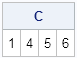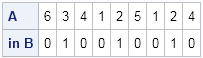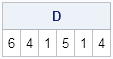The SAS/IML language has several functions for finding the unions, intersections, and differences between sets. In fact, two of my favorite utility functions are the UNIQUE function, which returns the unique elements in a matrix, and the SETDIF function, which returns the elements that are in one vector and not in another. Both functions return the elements in an ordered vector.

However, a recent question on the SAS/IML Support Community asked how to find the elements in one vector that are not in another while preserving the original order of the elements. As an example, consider the following vectors:

```proc iml; A = {6 3 4 1 2 5 1 2 4}; B = {2 3};```

The problem is how to find the elements in A that are not in B. If possible, we'd like to avoid using DO loops to iterate over the elements.

If you use the SETDIF function, the duplicate values in A are removed and the result is sorted:

```C = setdif(A,B); print C;```The output of the SETDIF function shows that the elements 1, 4, 5, and 6 are contained in A, but not in B. Although A contains two 1s and two 4s, that information is not included in the result.

If you need to preserve the original order of the data or you need to retain duplicate values, then you should use the ELEMENT function, which was introduced in SAS/IML 9.3. The ELEMENT function returns a matrix that is the same dimensions as A. The returned matrix is an "indicator matrix": a 1 in position (i, j) means that A[i, j] is an element of B, as shown by the following statements:

```E = element(A,B); print (A // E)[rowname={"A","in B"}];```The output shows that the second, fifth, and eighth elements of A are elements of B. If you want to preserve the original order of elements (and preserve duplicates), you can use the LOC function and the REMOVE function to eliminate those elements, as follows:

```D = remove(A,loc(element(A,B))); print D; /* you can also use subscripting: D = A[ loc(^element(A,B)) ]; */```The result lists the elements of A that are not in B, but preserves the order of the elements and any duplicate elements in the list.

Share